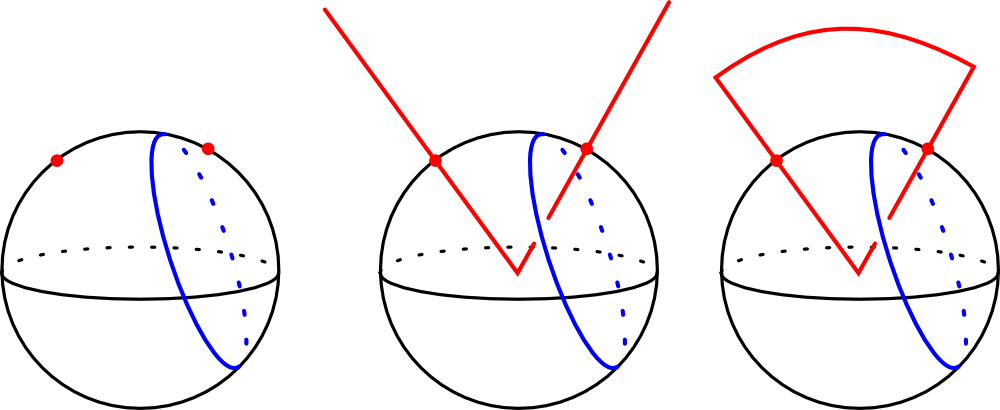# Clayton ShonkwilerIn our paper “Higher-dimensional linking integrals”, David Shea Vela-Vick and I found Gauss-type linking integrals in spheres of all dimensions.

Specifically, the Gauss linking integral computes the linking number between two parametrized closed curves in $$\mathbb{R}^3$$, and easily generalizes to compute the linking number between a $$k$$-cycle and an $$(n-k-1)$$-cycle in $$\mathbb{R}^n$$.

However, finding Gauss-type linking integrals even in the three-dimensional sphere is much trickier. In our paper, we found such integrals on all spheres by considering the $$n$$-sphere embedded in $$\mathbb{R}^{n+1}$$. By coning and capping, we tranform a $$k$$-cycle in the sphere into a $$(k+1)$$-cycle in $$\mathbb{R}^{n+1}$$ without changing the linking number. Then applying the Gauss integral in $$\mathbb{R}^{n+1}$$, pushing the cap off to infinity, and integrating out the cone direction yields a linking integral which is intrinsic to the sphere.

The cone-and-cap process is shown above on the 2-sphere, and the situation in higher dimensions is analogous.